## THE SQUARE-CUBE LAW & SCALING FOR RC SAILPLANES

This past summer I, unfortunately, found myself at the controls of someone else's large Sagitta XC (cross-country) after having been calibrated for years to fly my 2M Prodigy. The sailplane was high and downwind when I pushed the nose over to penetrate upwind. I had just reached what seemed to be a moderate and safe speed when suddenly the wings folded, and the glider tumbled to the ground in pieces – a fuselage and two wings. The problem was that I attempted to fly the large XC at speeds that appeared to be on the order of my small 2M. On another occasion, a friend of mine scaled a 2M Prodigy up to XC size. His initial reaction to the first few flights was that the 2M flew better – it "seemed" to fly faster than the XC. As further examples, a scale model sailplane looks faster than the full scale, a sparrow looks faster than a crow, and a Learjet looks faster than a DC-10.

So that you will not be easily deceived by what you see, it is worth going over why large aircraft appear to fly slower than small aircraft.

If we consider a class of aircraft of similar geometry and construction (for example, balsa and MonoKote or fiberglass and foam), the weight will scale as the cube of a characteristic length. This follows generally from mass being equal to density times volume. Taking the wing span as the characteristic length, we can therefore write: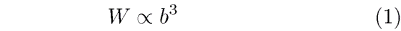Likewise for the wing area we have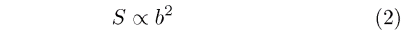These relations together form what is often called the "square-cube law," from which a wealth of useful information may be derived. For instance, these equations can be combined to give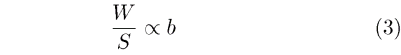Thus if a model is scaled up to twice its size, the wing loading will double if the construction materials are similar. This relationship can be used to estimate the weight of new designs based on old ones. It is only necessary to determine the proportionality constant. Also, from the equation for lift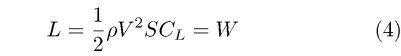we can obtain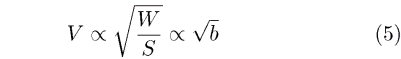This shows that if we increase the span and keep geometrically similar models with the same type of construction, then the speed at a given lift coefficient will increase with the square root of the wing span. It does not yet show why large aircraft look slower than small aircraft.

When an aircraft is flying against a blue sky, the only relative measure of length or size is the aircraft itself. Therefore, we can only base the speed on how long it takes the aircraft to cover some characteristic distance relative to its own size. If we take the wing span as this characteristic distance and call the time that it takes the aircraft to travel one wing span the "characteristic time," then we can write it as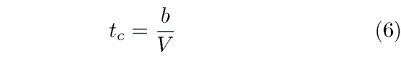From (4) we can than then write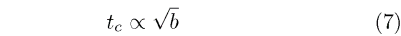Immediately we see that as the span increases so too does the time it takes to travel a distance of one wing span.

Finally, we can compare the "speeds" of a 2M and XC sailplane by forming the ratio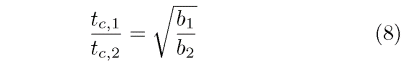If the wing span of the larger plane (denoted as 1) is twice the smaller (2), then the time it takes the larger plane to travel one wing span is 41% longer than the smaller. Although it is actually flying 41% faster (see equation (5)), it looks 41% slower. That is a big difference.

RC Soaring Digest Homepage